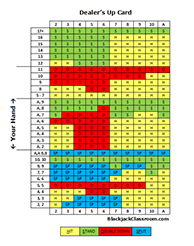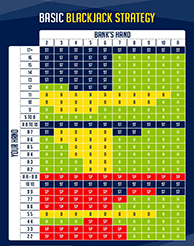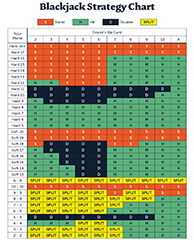# How Blackjack Odds are Broken DownOdds can be defined as the likelihood of an event or result occurring. This is the reason why the term is mostly used in gambling. In blackjack, you can only win if you understand the odds and how to use them in your favor. Use the information below to show you how you can use blackjack odds in your favor.

## How to Calculate Odds

Figuring out the probability of getting a specific card is easy. For example, let's say you want to get an ace of spades. You need to consider that a deck of cards has 52 cards and only one ace of spades. This leads to the conclusion that your probability is approximately 2%.

So how do you arrive at this percentage? It is simple, take 1 and divide it by 52, then multiply by 100. The calculation is 1/52 X 100 = 1.92%. This is the probability of getting an ace of spades in a deck of cards when you play blackjack.

Each outcome that has the probability of occurring in a game of blackjacks will vary from 0 to 100 percent. With this in mind, you will know if you have a high or low probability of an event occurring. This means you can also gauge the likelihood of an event not taking place during the game.

For instance, if your chances of getting a card are 20%, it means you have an 80% chance of not getting the card. The same principle can be used to calculate your probability of winning or losing a game of blackjack.

## Practical Example of Blackjack OddsLet's say you want to find out the odds of drawing an ace of diamonds. Since it is only one card in a deck, the remaining 52 cards will be different. The odds in such a scenario is 51 to 1. These are very low odds.

In a game of blackjack, there are two terms that you should not ignore. The first term is a negative or positive win rate. It is used to describe the probability you have at any point in the game.

The second term is known as true odds. It is a term commonly used by professional gamblers. They use it when a wager pays out at the rate the probability percentage had suggested.

## Goals for Using Blackjack OddsYou can only win at blackjack by surpassing the dealer's total without hitting more than 21 points. The two main options are standing and hitting. These options decide whether you win or lose.

You will also need to know your busting odds to get an idea of your current position. This helps you understand the logic behind any suggested moves. For example, let's say you have 11 or less in your hand. In this case, your busting odds are 0%.

If you have anything between 12 and 14, your busting odds are 31% to 56%. This means your busting odds increase as your total hand increases. If the total you have is 19 or more, avoid hitting. The odds of busting are more than 85%.

The likelihood of getting the highest paying combination in blackjack has been calculated. It states getting a 10-value card or an ace from the initial deal when you play a single deck game is 4% likely. The same applies when you play a two-deck game.